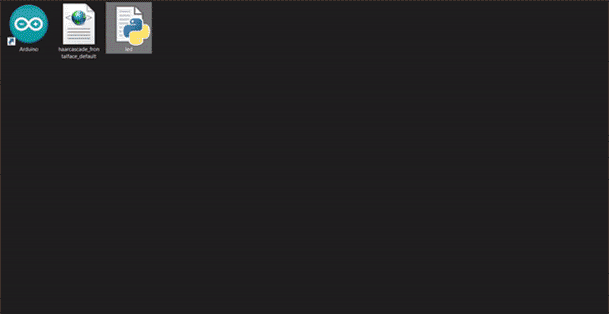L O A D I N G## Image processing with Python and executing commands with Arduino

2022

### Python:

Step 2 Install the pip
Enter the following phrase to install pip in the command line environment

pip install pip

Step 3 Install an IDE code editor I suggest Visual Studio Code
So far we have Python and pip to install libraries in Python and a code editor
Now we need to install the libraries needed for this project.
Step 4 To install opencv, which is for image processing, install the following command with the help of pip in the command line environment.

pip install opencv-python

Step 5 To install the PySerial library and connect to the Arduino, download the file and unzip the contents of the file in any folder such as "C: \ pyserial-3.5". Download the PySerial file.
Location is important because we need to go to this location and install pySerial.
Now, run Command Prompt in Administrator mode and go to the folder where pySerial is extracted.

cd C:\pyserial-3.5

To install pySerial, type the following command on the command line and press Enter.

python setup.py install

Or you can install with the command line.

pip install pyserial

Successfully installed.

Step 6 To install the time library, enter the following command in the command line environment for the delay functions.

pip install time

Step 7 Enter the code editor and create a page with the py extension.
Step 8 Import the installed libraries at the top of the page.

import cv2
import serial #for Serial communication
import time #for delay functions

Step 9 Create Serial port object called arduino Serial Data.

arduino = serial.Serial('com4',9600)

Step 10 Pause for 2 seconds to communicate

time.sleep(2)

Step 11 Use the following code to activate the webcam.

cap = cv2.VideoCapture(0)

Step 13 We use a loop to turn photos into video

while(True):

Step 14 Capture frame by frame

Step 15 Our operations on the frame come here

gray = cv2.cvtColor(frame, cv2.COLOR_BGR2GRAY)

Step 16 Detect faces in the image

gray,
scaleFactor=1.1,
minNeighbors=5,
minSize=(30, 30)
#flags = cv2.CV_HAAR_SCALE_IMAGE )

Step 17 Use the code below to display the number of faces detected

print("Found {0} faces!".format(len(faces)))

Step 18 We check by making a condition If the face is identified, send the number 1 to Arduino, and if not, send the number 0.

if (format(len(faces)) == '1'):
arduino.write(b'1')

if (format(len(faces)) == '0'):
arduino.write(b'0')

Step 19 Draw a rectangle around the faces

for (x, y, w, h) in faces:
cv2.rectangle(frame, (x, y), (x+w, y+h), (0, 255, 0), 2)

Step 20 Start the program by pressing the q key

cv2.imshow('frame', frame)
if cv2.waitKey(1) & 0xFF == ord('q'):
break

When everything done, release the capture

cap.release()
cv2.destroyAllWindows()

### Arduino:

Step 2 Connect the Arduino board to the system

const int led=13;
int value=0;
void setup()
{
Serial.begin(9600);
pinMode(led, OUTPUT);
digitalWrite (led, LOW);
}

void loop()
{
while (Serial.available())
{